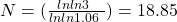Time Value of Money | IFT World
IFT Notes for Level I CFA® Program
IFT Notes for Level I CFA® Program

# Part 4

## 11. Solving for Interest Rates, Growth Rates, and Number of Periods

### 11.1. Solving for Interest Rates and Growth Rates

An interest rate can also be considered a growth rate. We can compute the rate using the formula method or the calculator method.

Example:

A $100 deposit today grows to$121 in 2 years. What is the interest rate? Use both the formula and the calculator method.

Using the formula 100(1+r) 2 = 121. Therefore r = 0.1 or 10%

Using the calculator method, inputs to the calculator are

PV = -$100 (When we enter both PV and FV, they should be given opposite signs to avoid a calculator error.) FV =$121

N = 2

PMT = 0

CPT I → I = 10%

Example:

The population of a small town is 100,000 on 1 Jan 2000. On 31 December 2001 the population is 121,000. What is the growth rate?

Inputs to the calculator are

PV = -$100,000 FV =$121,000

N = 2

PMT = 0

CPT I → I = 10%

Example:

You invest $900 today and receive a$100 coupon payment at the end of every year for 5 years. In addition, you receive $1,000 and the end of year 5. What is the interest rate? Inputs to the calculator are PV = -$900

FV = $1,000 N = 5 PMT = 100 CPT I → I = 12.83% ### 11.2. Solving for the Number of Periods Similarly, we can determine the number of periods given other information such as future value, present value and interest rate. Example: You invest$2,500. How many years will it take to triple the amount given that the interest rate is 6% per annum compounded annually? Use both the formula and the calculator method.

Formula Method:

FV = PV (1+r)N
7500 = 2500 (1+0.06)N
1.06N= 3
Nx(lnln1.06) = ln ln 3Calculator Method:

Using the calculator: I/Y = 6%, PV = $2,500, PMT = 0, FV = -$7,500, CPT N = 18.85.

## 12. Solving for the Size of Annuity Payments

Given the number of periods, interest rate per period, present value, and future value, it is easy to solve for the annuity payment amount. This concept can be applied to mortgages and retirement planning. Consider the following example.

Example:

Freddie bought a car worth $42,000 today. He was required to make a 15% down payment. The remainder was to be paid as a monthly payment over the next 12 months with the first payment due at t = 1. Given that the interest rate is 8% per annum compounded monthly, what is the approximate monthly payment? Loan amount = 85% of$42,000 = 0.85 x 42,000 = $35,700 PV =$35,700

N = 12

I/Y = 8/12%

### 13.1. The Cash Flow Additivity Principle

Amounts of money indexed at the same point in time are additive. For example, if you have the following cash flows:

You cannot simply add these three numbers. You have to take each of these numbers and bring them to a particular point in time. Let’s say that we find the present values at time zero for each of these cash flows. According to this principle, these present values that are all indexed to time zero can be added.

LIVE WEEKEND CLASSES *For 2024 Exam candidates*
This is default text for notification bar